# Corresponding sides

Corresponding sides
Go back to  'Triangles'

In geometry, finding the congruence and similarity involves comparing corresponding sides and corresponding angles of the polygons.

Corresponding sides are the sides that are in the same position in different 2-dimensional shapes.

Consider the 2 quadrilaterals ABCD and PQRS in the image below.

AB corresponds to PQ, BC corresponds to QR, CD corresponds to RS and DA corresponds to SP.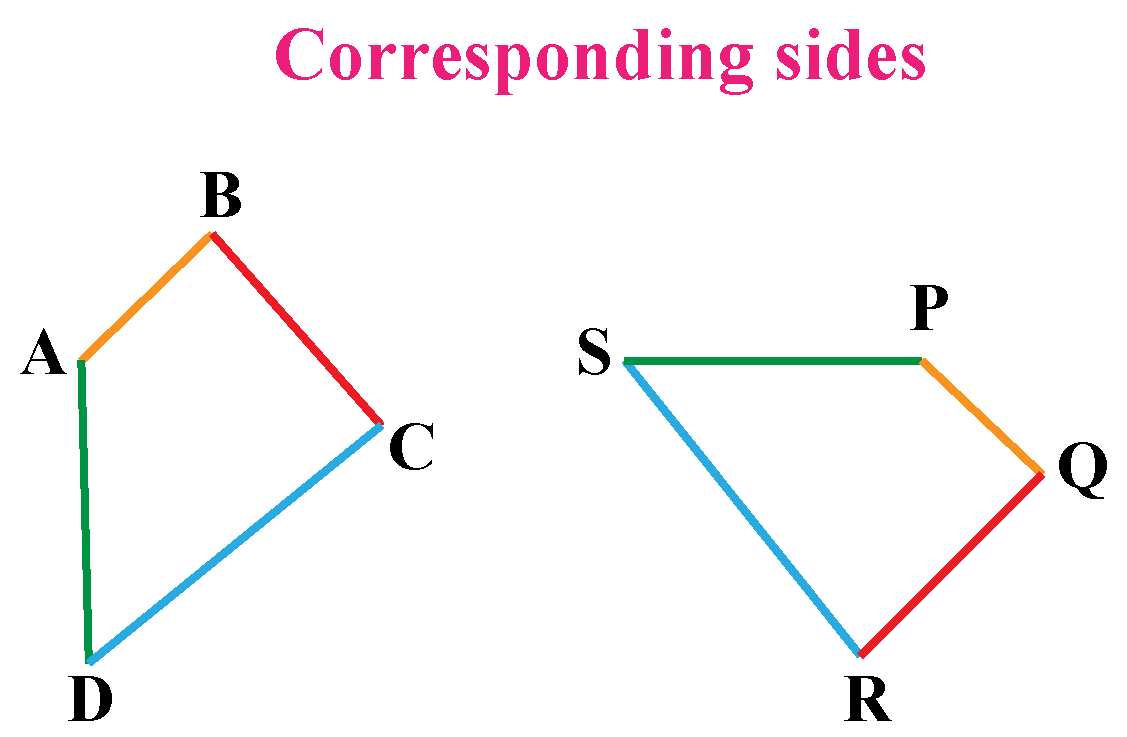In this mini-lesson, we will learn about the corresponding sides definition, similar triangles, similar right triangles, and congruent by knowing the interesting facts around them.

## Lesson Plan

 1 What are Corresponding Sides of Triangles? 2 Tips and Tricks 3 Important Notes on Corresponding Sides 4 Solved Examples on Corresponding Sides 5 Interactive Questions on Corresponding Sides

## What are Corresponding Sides of Triangles?

### Corresponding Sides Definition

Corresponding sides of a triangle are the sides that are in the same position in different triangles.

Consider two triangles ABC and LMN in the image below. Start with any side, say AB in the $$\triangle ABC$$.

Locate the similar side corresponding to this AB.

We find with respect to its position, that LM is the corresponding side to AB in $$\triangle LMN$$.

Likewise, we identify that BC in $$\triangle ABC$$ is the corresponding side to MN in $$\triangle LMN$$ and

CA is the corresponding side to NL.

Thus locating one side corresponding to the other helps us to identify the other corresponding sides of the two triangles.

### Congruent Triangles vs Similar Triangles

The congruent triangles are different from similar triangles considering the aspect of corresponding sides.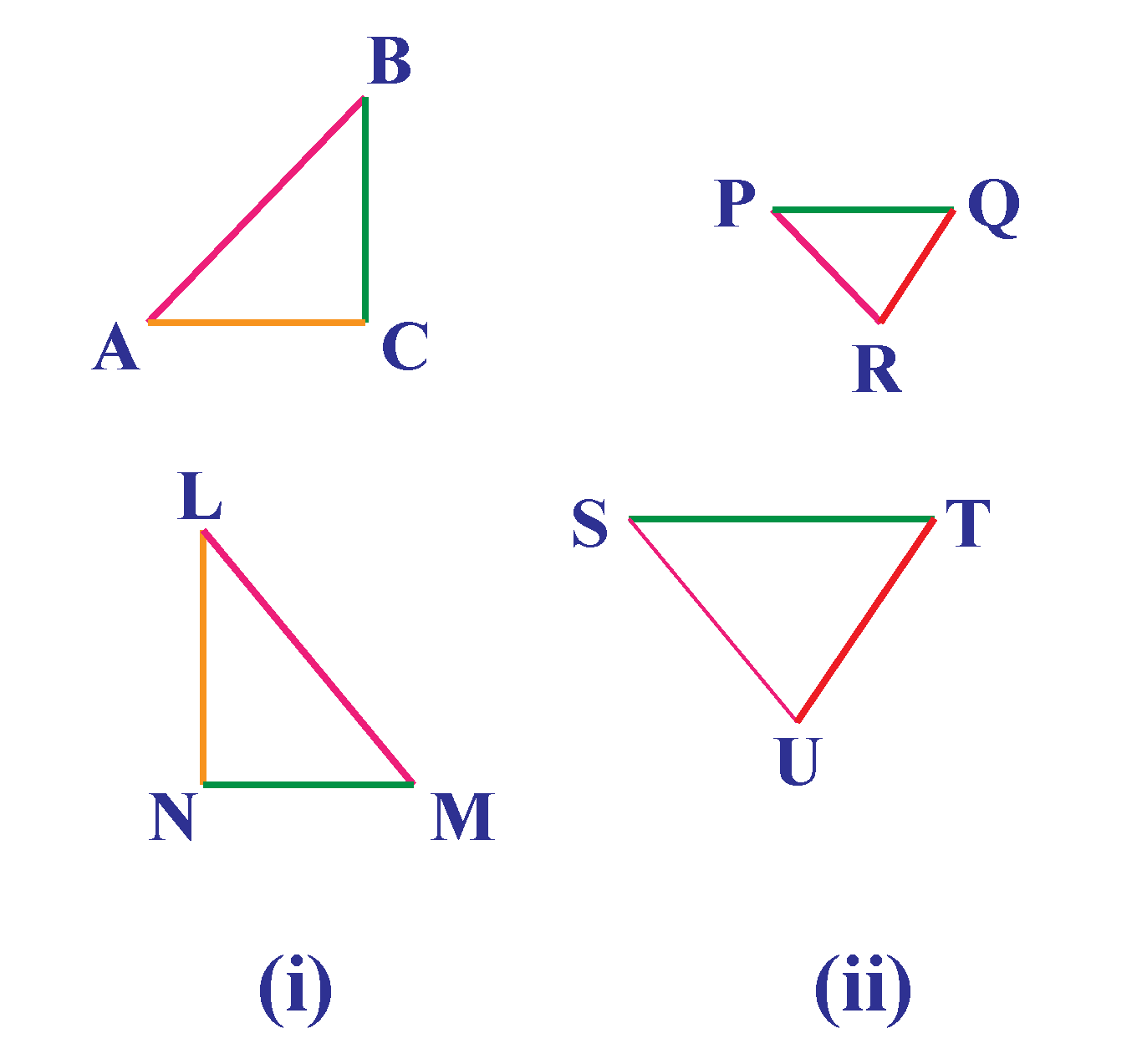Congruent Triangles Similar Triangles
Two triangles are congruent if they have all their corresponding angles and sides equal.    Two triangles are similar if they have all their corresponding angles equal and their corresponding sides are in the same ratio.

In $$\triangle$$ABC and $$\triangle$$LMN ,

(1) AB = LM, BC = MN, and AC = LN.

(2) $$\angle$$ A = $$\angle$$ M, $$\angle$$ B = $$\angle$$ L, $$\angle$$ C = $$\angle$$N

$$\therefore \triangle$$ ABC $$\cong \triangle$$ LMN

In $$\triangle$$PQR and $$\triangle$$SUT,

(1)PQ $$\propto$$ ST, QR $$\propto$$ TU, and PR $$\propto$$ SU

(2) $$\angle$$ P = $$\angle$$ S, $$\angle$$ Q = $$\angle$$ T, $$\angle$$ R = $$\angle$$ U

$$\therefore \triangle$$ PQR $$\simeq \triangle$$ STU

## How Do You Know If The Corresponding Sides Are Proportional?

If the two shapes are similar, then their corresponding sides are proportional.

### Similar Triangles

If an angle of one triangle is equal to the corresponding angle of another triangle and the lengths of the sides including these angles are in proportion, the triangles are similar.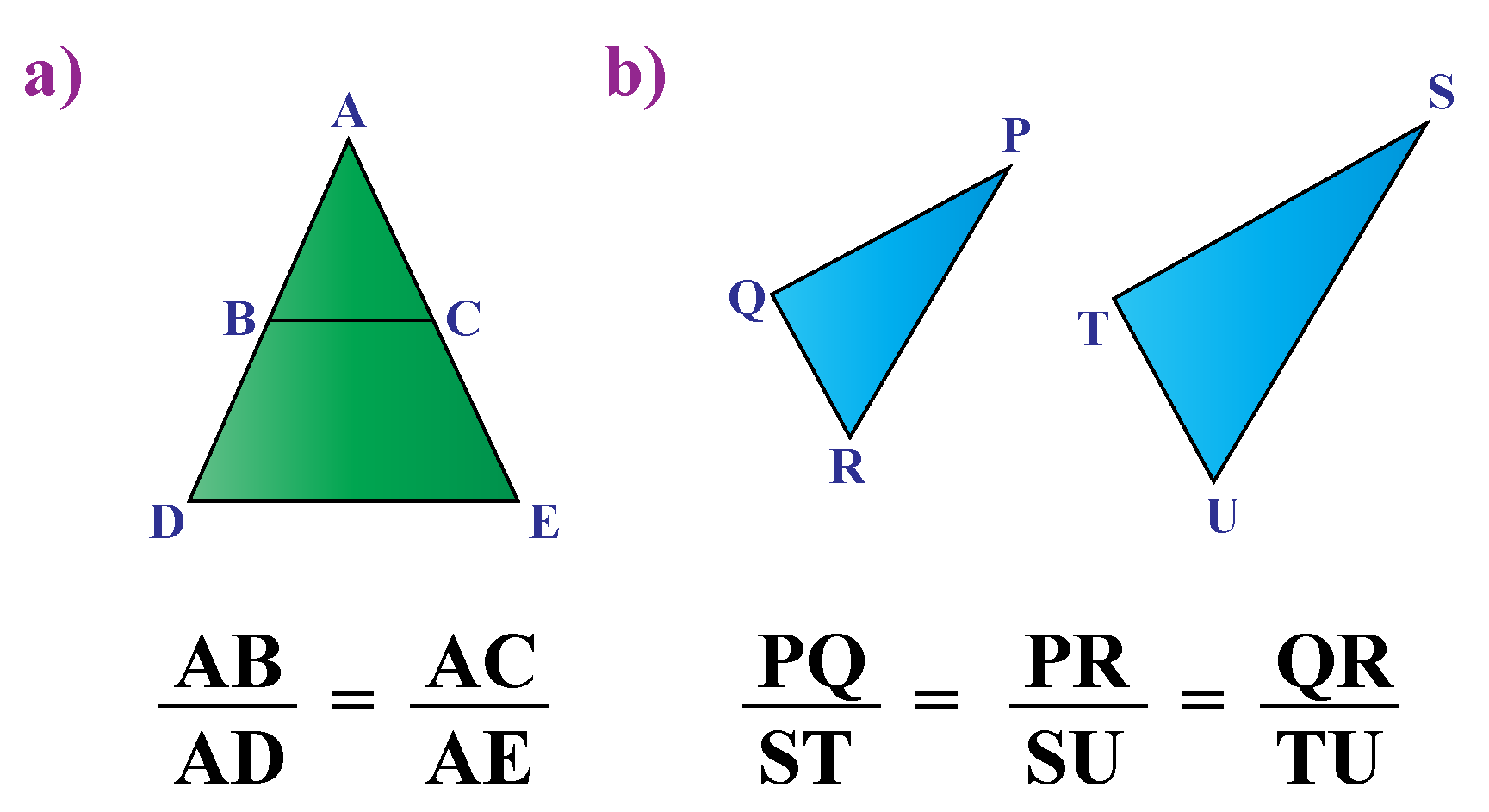Consider a)  $$\triangle \text{ABC} \simeq \triangle \text{ADE}$$

$\dfrac{\text{AB}}{\text{AD}} = \dfrac{\text{AC}}{\text{AE}}$

$\text{AB}\times \text{AE} = \times{AD}\times \text{AC}$

Consider b) $$\triangle \text{PQR} \simeq \triangle \text{STU}$$

$\dfrac{\text{PQ}}{\text{ST}} = \dfrac{\text{PR}}{\text{SU}} =\dfrac{\text{QR}}{\text{TU}}$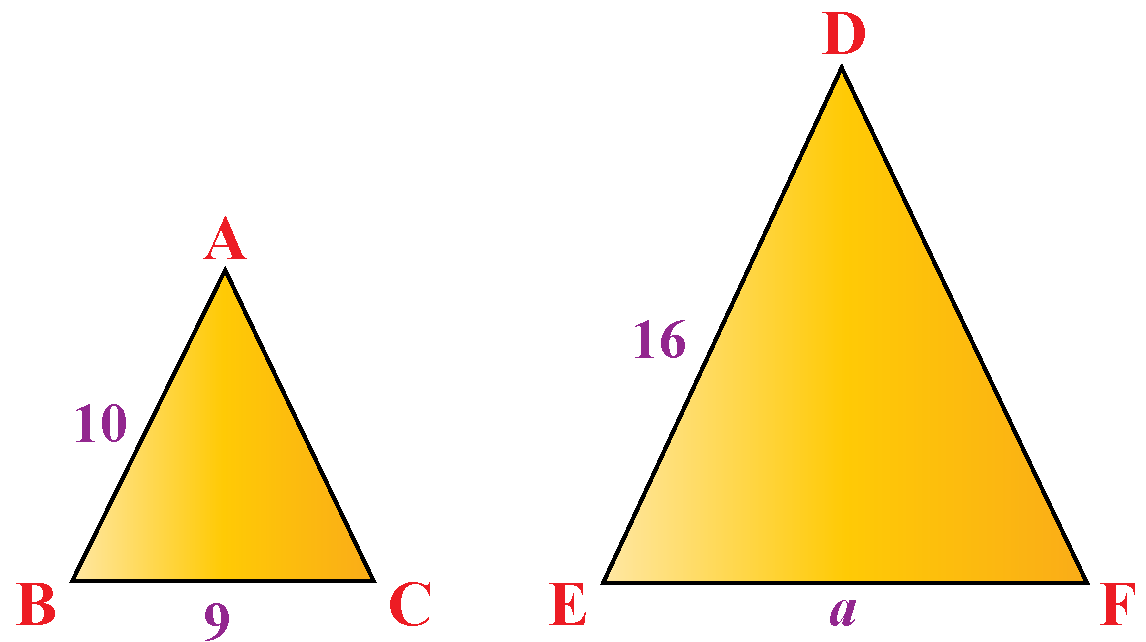Consider triangles ABC and DEF.

If two triangles are similar, then their corresponding sides are proportional.

\begin{align}\dfrac{\text{AB}}{\text{DE}} &= \dfrac{\text{BC}}{\text{EF}}\\\\\dfrac{10}{16} &= \dfrac{9}{a}\\\\ 10 \times a &= 16 \times 9\\\\ a&= \dfrac{(16\times 9)}{10}\\\\ &=\dfrac{144}{10}\\\\ &= 14.4\end{align}

In the following simulation, the two triangles ABC and PQR are similar.

Drag the points A, B, C.

What can you notice about the triangles?

Verify for yourself by sliding the points that the equivalent sides are always proportional.

Thus we conclude that if $$\triangle \text{ABC} \simeq \text{PQR}$$, then we say that the corresponding sides are proportional and the angles are equal.

 $$\dfrac{\text{AB}}{\text{PQ}} = \dfrac{\text{BC}}{\text{QR}} =\dfrac{\text{CA}}{\text{RP}} = k$$

where k is the equivalent ratio or the trigonometric ratio.Tips and Tricks
1. Locate one corresponding side and the others will fall in sequence.
2. Finding the corresponding sides helps us find the corresponding angles as well, as the angle is subtended between two sides.

## Corresponding Sides in Right Triangles

If the lengths of the hypotenuse and a leg of one right triangle are proportional to the corresponding parts of the other right triangle, then the triangles are similar.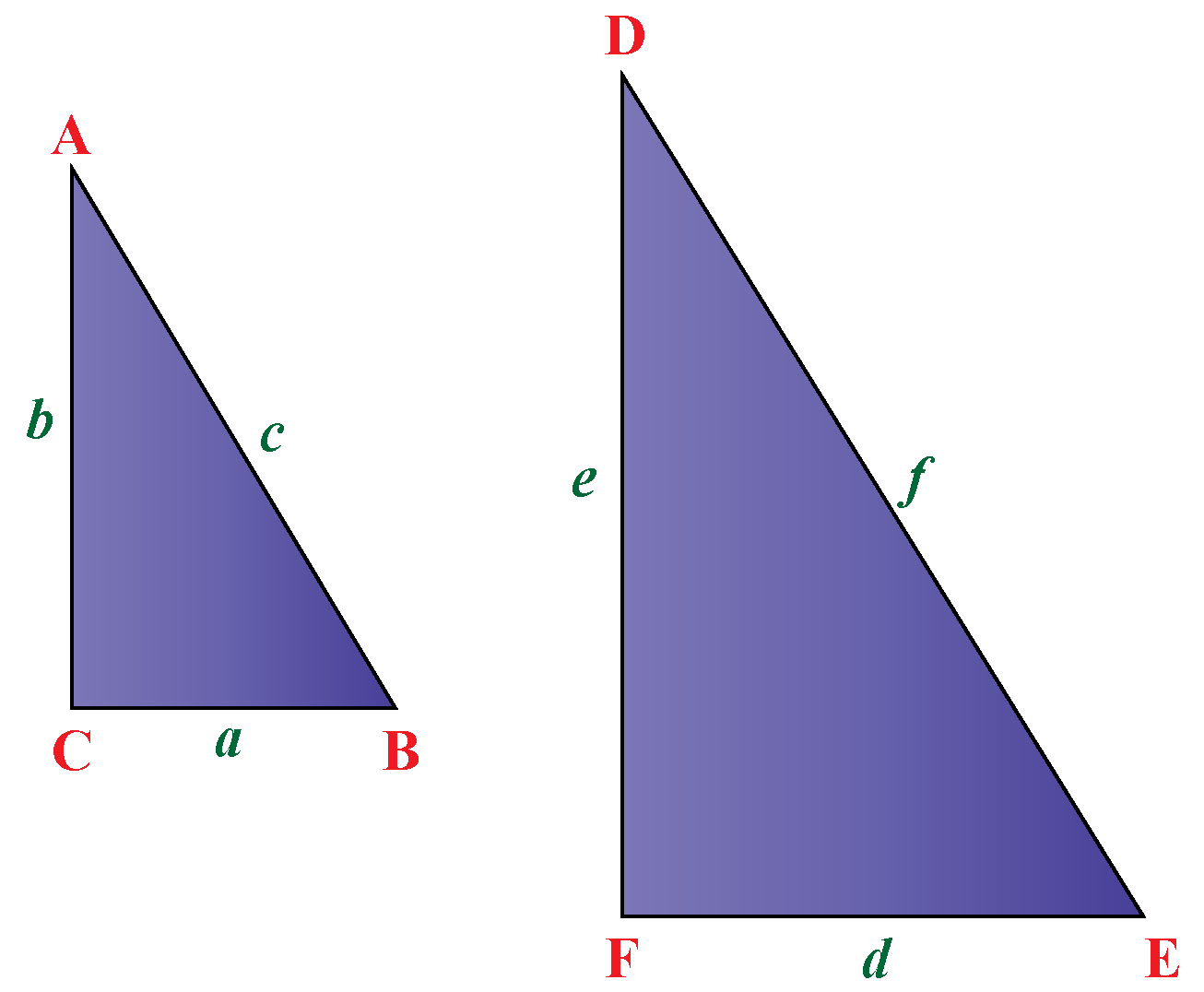$\dfrac{\text{The shortest side of the small triangle}}{\text{The shortest side of the large triangle}}\\=\dfrac {\text{The longest side of the small triangle}} {\text{The longest side of the large triangle}}\\= \dfrac{\text{Hypotenuse of small triangle}}{\text{Hypotenuse of the large triangle}}$

$\dfrac{\text{a}}{\text{d}}=\dfrac {\text{b}} {\text{e}}= \dfrac{\text{c}}{\text{f}}$

## Solved Examples

 Example 1

Brandon wants to check if the two triangles are similar. Can you help him?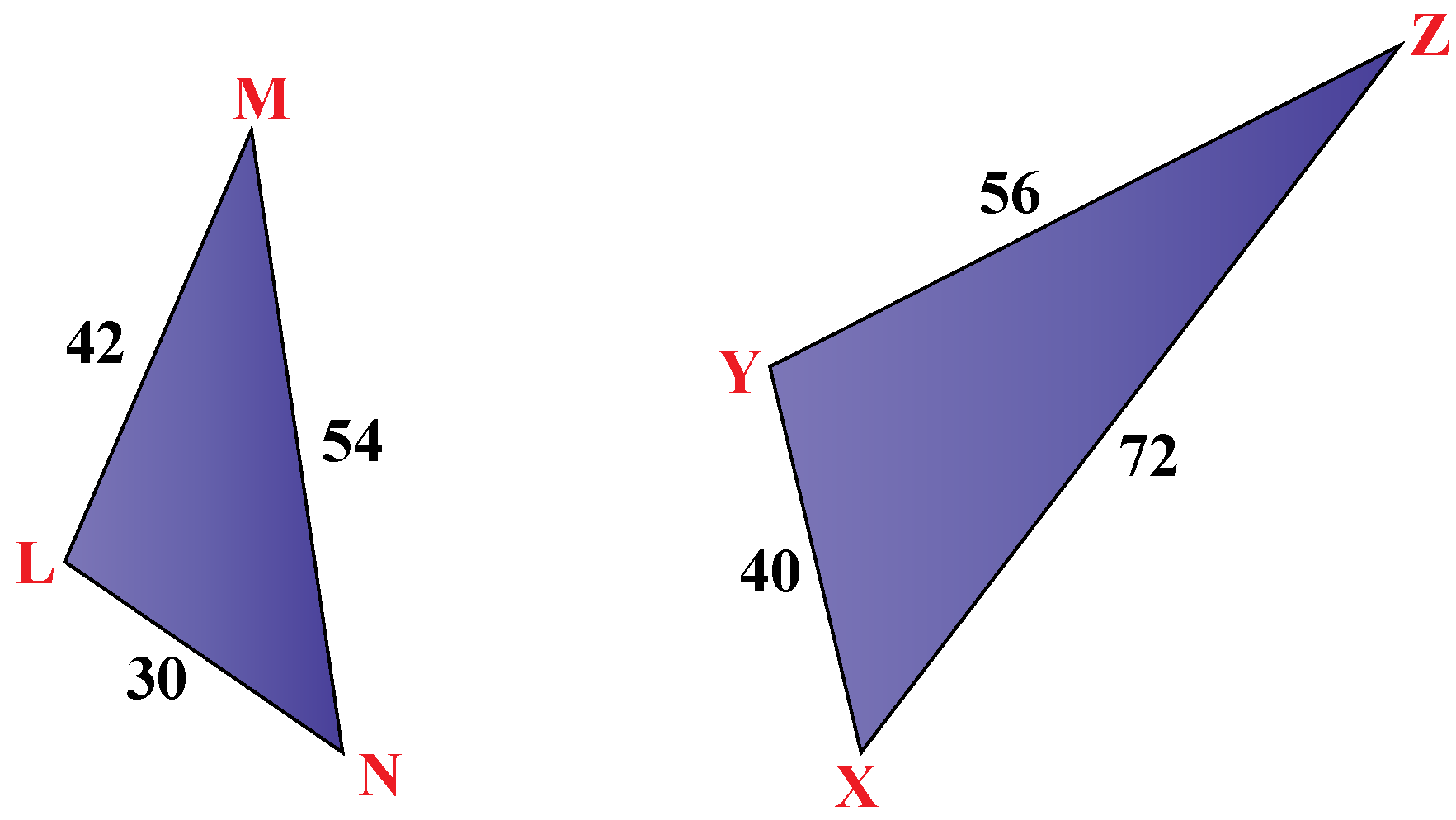Solution

To determine if the triangles are similar we need to check if the sides are proportional.

Identify the corresponding sides in $$\triangle$$LMN and $$\triangle$$XYZ.

$$\dfrac{LN}{XY} = \dfrac{30}{40} = \dfrac{30\div 10}{40\div 10} = \dfrac{3}{4}$$

$$\dfrac{LM}{YZ} = \dfrac{42}{56}= \dfrac{42\div 14}{56\div 14} = \dfrac{3}{4}$$

$$\dfrac{MN}{ZX} = \dfrac{54}{72} = \dfrac{54\div 9}{72\div 9} = \dfrac{3}{4}$$

We find that the corresponding sides are proportional to each other.

 $$\therefore$$ The two triangles are similar.
 Example 2

Ria was arranging a few 2-dimensional shapes while she was constructing a math puzzle. She found 2 triangles arranged in the way shown below. On observation, she found that the triangles are similar. She wanted to know which are the corresponding sides of these triangles. How can you help her?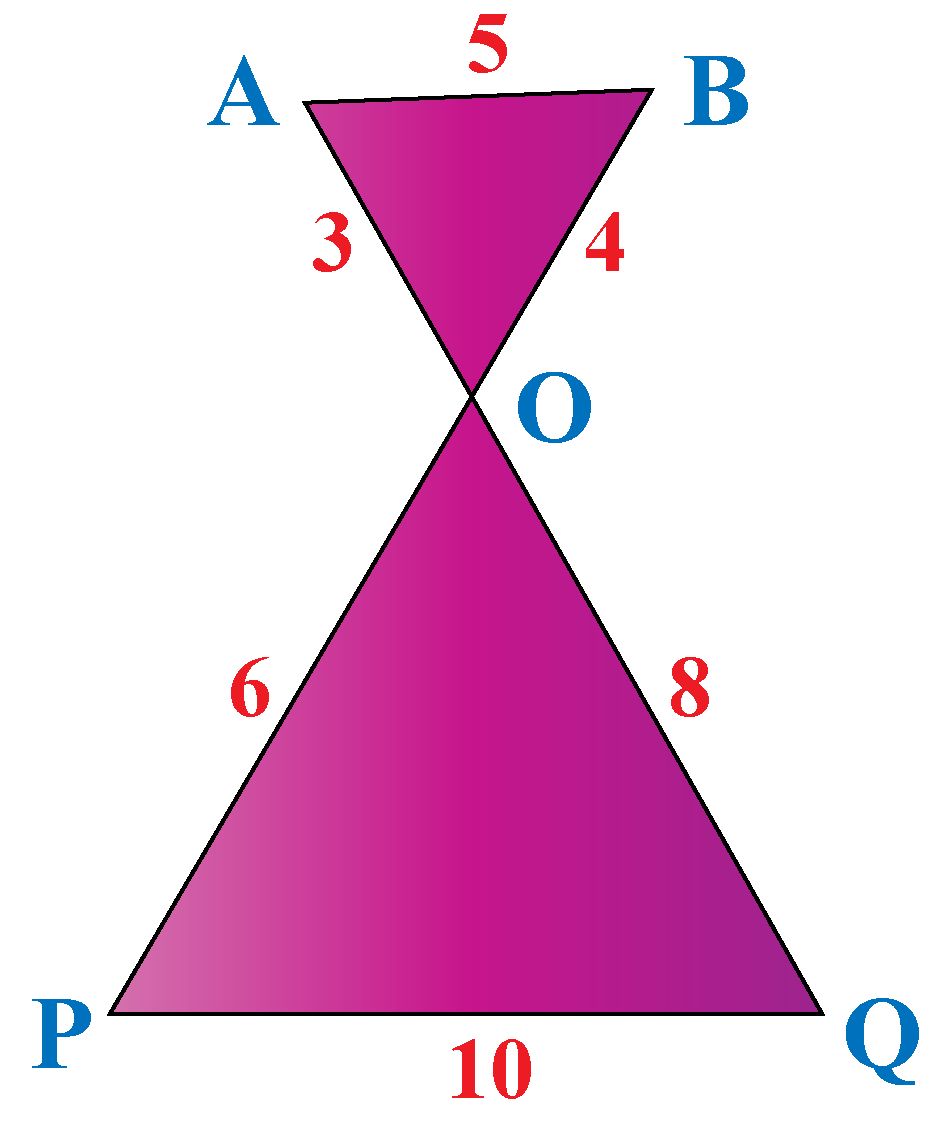Solution

AOB and POQ are the two triangles.

Known that they are similar.

If the triangles are similar, the sides are proportional.

Check which two sides form the equal proportion.

We find that $$\dfrac{3}{6}=\dfrac{1}{2}\\\dfrac{4}{8}=\dfrac{1}{2}\\\dfrac{5}{10}=\dfrac{1}{2}$$

$$\dfrac{OA}{OP}=\dfrac{OB}{OQ}=\dfrac{AB}{PQ}=\dfrac{1}{2}$$

 $$\therefore$$ OA corresponds to OP, OB corresponds to OQ and AB corresponds to PQ.
 Example 3

Marc has made a paper fold for his project. He wants to know the side 'f'  if $$\triangle$$ OPN and $$\triangle$$ OQM are similar. How can you help him?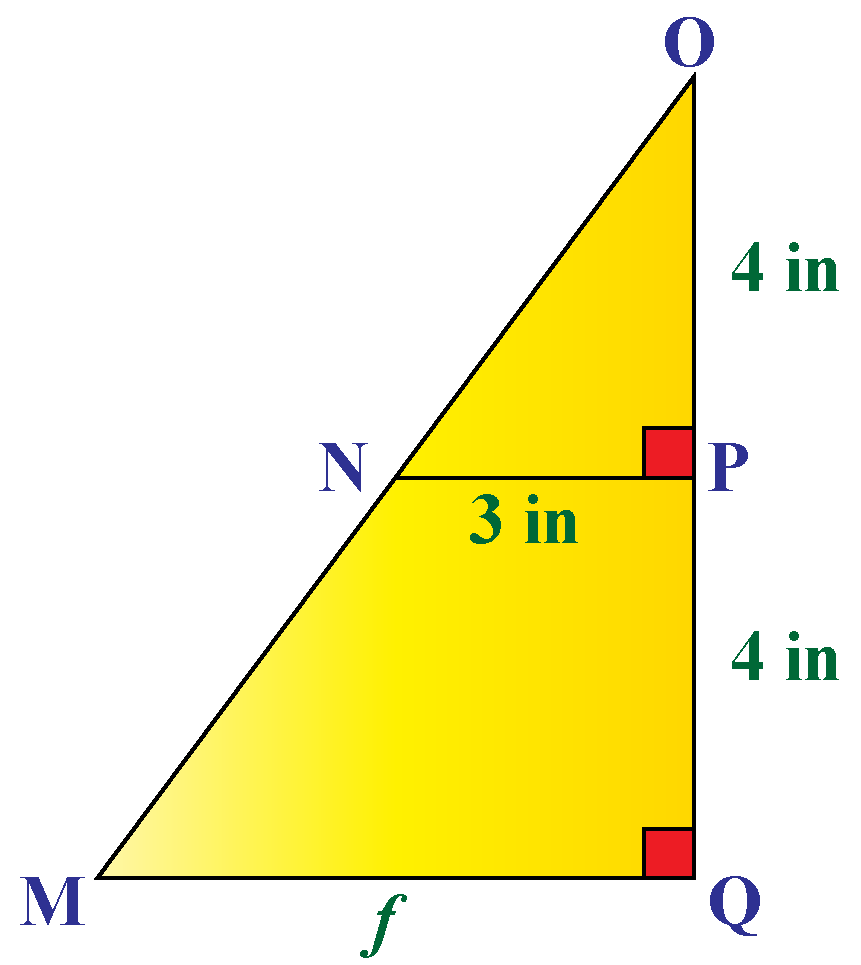Solution

OP = 4 inches

OQ = 8 inches

Since the two triangles are similar, the ratios of the lengths of their corresponding sides are equal.

\begin{align}\dfrac{\text{4}}{\text{8}}&=\dfrac {\text{3}} {\text{f}}\\ 4 \times\text{f} &= 3\times 8 \\\text{f}&= \dfrac{24}{4}\\ \text{f} &= 6 \text{ inches}\end{align}

 $$\therefore$$ the unknown side $$\text{f} = 6 \text{ inches}$$

## Interactive Questions

Here are a few activities for you to practice. Select/Type your answer and click the "Check Answer" button to see the result.

## Let's Summarize

The mini-lesson targeted the fascinating concept of corresponding sides. The math journey around corresponding sides starts with what a student already knows, and goes on to creatively crafting a fresh concept in the young minds. Done in a way that not only it is relatable and easy to grasp, but also will stay with them forever. Here lies the magic with Cuemath.

At Cuemath, our team of math experts is dedicated to making learning fun for our favorite readers, the students!

Through an interactive and engaging learning-teaching-learning approach, the teachers explore all angles of a topic.

Be it worksheets, online classes, doubt sessions, or any other form of relation, it’s the logical thinking and smart learning approach that we, at Cuemath, believe in.

## 1. What are similar triangles?

Two triangles are said to be similar if their corresponding angles are equal and the corresponding sides are proportional.

## 2. Are corresponding sides equal?

Two congruent triangles have their corresponding sides equal. Two similar triangles have their corresponding sides proportional.

More Important Topics
Numbers
Algebra
Geometry
Measurement
Money
Data
Trigonometry
Calculus